# C++ Program to Interchange the Digits of a Number

In this article, you will learn and get code to interchange the digits of a number entered by the user at run-time in C++. Here is the list of programs available in this article:

## Interchange the first and last digit of a number in C++

To interchange the first and last digits of a number in C++ programming, you have to ask the user to enter the number. And then interchange its first and last digit as shown in the program given below:

The question is: write a program in C++ to interchange the first and last digits of a number. Here is its answer:

```#include<iostream>
using namespace std;
int main()
{
int num, rem, temp, rev=0, noOfDigit=0;
int noOfDigitTemp, revNum, remTemp;
cout<<"Enter the Number: ";
cin>>num;
temp = num;
while(temp>0)
{
temp = temp/10;
noOfDigit++;
}
if(noOfDigit<2)
{
cout<<"\nIt is a Single-digit Number!";
cout<<"\nTry again with a Number with Two or More Digits!";
}
else if(noOfDigit==2)
{
temp = num;
while(temp>0)
{
rem = temp%10;
rev = (rev*10)+rem;
temp = temp/10;
}
cout<<"\nFirst and Last Digit Interchanged Successfully!";
cout<<"\n\nNew Number = "<<rev;
}
else
{
temp = num;
while(temp>0)
{
rem = temp%10;
rev = (rev*10)+rem;
temp = temp/10;
}
revNum = rev;
rev = 0;
temp = num;
noOfDigitTemp = noOfDigit;
while(temp>0)
{
remTemp = revNum%10;
if(noOfDigitTemp==noOfDigit)
{
rem = temp%10;
rev = (rev*10)+rem;
}
else if(noOfDigitTemp==1)
{
rem = temp%10;
rev = (rev*10)+rem;
}
else
{
rev = (rev*10)+remTemp;
}
temp = temp/10;
revNum = revNum/10;
noOfDigitTemp--;
}
cout<<"\nFirst and Last Digit Interchanged Successfully!";
cout<<"\n\nNew Number = "<<rev;
}
cout<<endl;
return 0;
}```

This program was built and runs under the Code::Blocks IDE. Here is its sample run: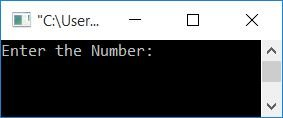Now supply any number, say 1234, and press ENTER to interchange the first (1) and last (4) digits of this number. After exchanging the first and last digits, print the new number as shown in the snapshot of the sample run given below: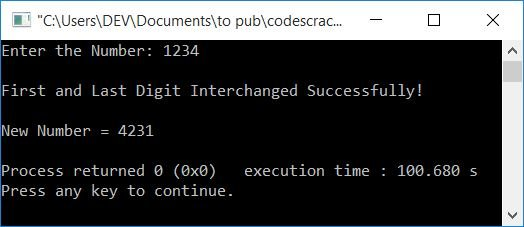Here is another sample run with user input 12, a two-digit number: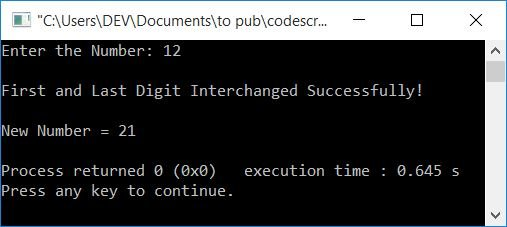Here is one more sample run with user input 3, a single-digit number: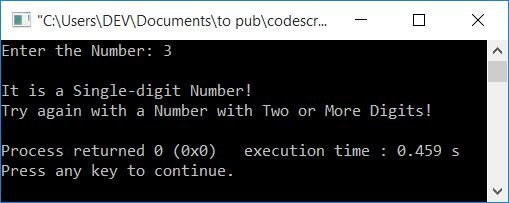What happens if the user enters a number that ends in 0? Or if the user enters a number that has 0 as its last digit, then the above program produces the following output in this case: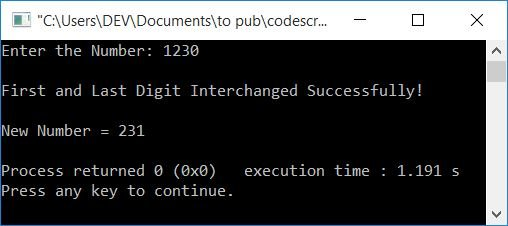To solve this problem, we have another program for you. This program does the same job, but using an array. Let's have a look at the program given below.

### In C++, use an array to swap the first and last digits

This program does the same job, which is to interpolate the first and last digits of a number given by the user using an array.

```#include<iostream>
using namespace std;
int main()
{
int num, rem, temp, rev=0, noOfDigit=0, arr, i;
cout<<"Enter the Number: ";
cin>>num;
temp = num;
while(temp>0)
{
temp = temp/10;
noOfDigit++;
}
temp = num;
while(temp>0)
{
rem = temp%10;
rev = (rev*10)+rem;
temp = temp/10;
}
for(i=0; i<noOfDigit; i++)
{
rem = rev%10;
arr[i] = rem;
rev = rev/10;
}
if(noOfDigit==1)
{
cout<<"\nIt is a Single-digit Number!";
cout<<"\nTry again with a Number with Two or More Digits!";
}
else if(noOfDigit==2)
{
temp = arr;
arr = arr;
arr = temp;
cout<<"\nFirst and Last Digit Interchanged Successfully!";
cout<<"\n\nNew Number = "<<arr<<arr;
}
else
{
i=0;
temp = arr[i];
arr[i] = arr[noOfDigit-1];
arr[noOfDigit-1] = temp;
cout<<"\nFirst and Last Digit Interchanged Successfully!";
cout<<"\n\nNew Number = ";
for(i=0; i<noOfDigit; i++)
cout<<arr[i];
}
cout<<endl;
return 0;
}```

Here is its sample run with user input, 1230: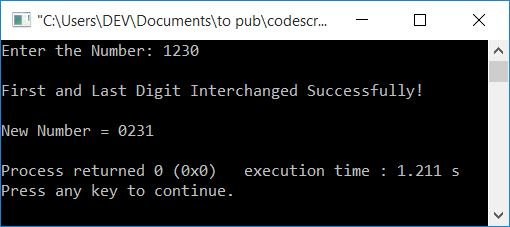## Interchange any two digits of a number in C++

This program is created to interchange any two digits of a number. Any two-digit number allows you to swap the digit at position 2 with the digit at position 5, say:

For example, if a user enters the number 23706 and wishes to swap the digit in the second position (3) with the digit in the fifth position (6), then the new number, after changing the required digit, will be 26703.

```#include<iostream>
using namespace std;
int main()
{
int num, posFirst, posSecond;
int rem, temp, rev=0, noOfDigit=0, arr, i;
cout<<"Enter the Number: ";
cin>>num;
temp = num;
while(temp>0)
{
temp = temp/10;
noOfDigit++;
}
if(noOfDigit==1)
{
cout<<"\nIt is a Single-digit Number!";
cout<<"\nTry again with a Number with Two or More Digits!";
}
else
{
cout<<"\nInterchange the Digit at Position: ";
cin>>posFirst;
cout<<"With Digit at Position: ";
cin>>posSecond;
}
if(posFirst>noOfDigit || posSecond>noOfDigit)
cout<<"\nInvalid Position!";
else
{
temp = num;
while(temp>0)
{
rem = temp%10;
rev = (rev*10)+rem;
temp = temp/10;
}
for(i=0; i<noOfDigit; i++)
{
rem = rev%10;
arr[i] = rem;
rev = rev/10;
}
i=0;
temp = arr[posFirst-1];
arr[posFirst-1] = arr[posSecond-1];
arr[posSecond-1] = temp;
cout<<"\nDigits Interchanged Successfully!";
cout<<"\n\nNew Number = ";
for(i=0; i<noOfDigit; i++)
cout<<arr[i];
}
cout<<endl;
return 0;
}```

Here is its sample run with user input, 123403 as a number, and 3 and 5 as digit positions to interchange: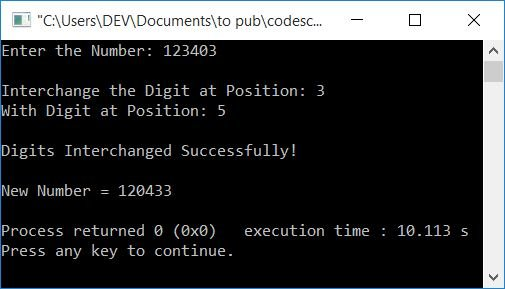#### The same program in different languages

C++ Quiz

« Previous Program Next Program »